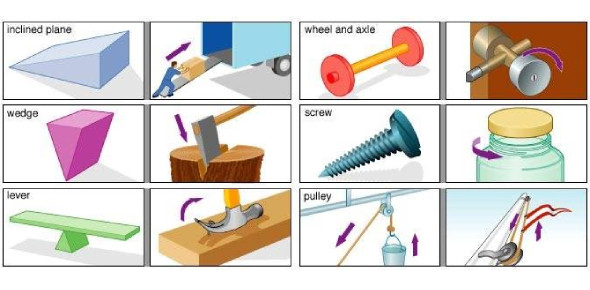# Physics Quiz: Motion, Simple Machines, And Waves

20 Questions | Attempts: 424
ShareSettingsAre you ready for this motion, simple machines, and waves physics quiz? One of the basic rules of physics is that for every action, there is a reaction. Simple machines have been in use for the longest time ever in the building of the pyramids. Power is the rate at which work is done, and machines increase this rate by a significant amount. Take up this quiz and get to see how much you know about power and motion.

• 1.
_____________ is the lowest point between each crest of a wave.
• A.

Trough

• B.

Wavelength

• C.

Compression

• D.

None of these

• 2.
What is the unit for power?
• A.

Joule

• B.

Newton

• C.

Kelvin

• D.

Watt

• 3.
Referring to one of Newton’s Laws, for every action, the reaction is ______________________
• A.

Equal and opposite

• B.

Increased significantly

• C.

Equal and in the same direction

• D.

None of these

• 4.
What is power?
• A.

The rate at which work is done

• B.

The work that is done

• C.

The strength of someone or something

• D.

The mass of an object

• 5.
As the wavelength increases, the frequency
• A.

Decreases

• B.

Increases

• C.

Stays the same

• D.

Increases and then decreases

• 6.
How is work calculated?
• A.

Power times force

• B.

Power divided by distance

• C.

Force times distance

• D.

Power multiplied by the distance

• 7.
___________ is a unit that measures force.
• A.

Meter

• B.

Work

• C.

Watt

• D.

Newton

• 8.
A ___________________ is a push or pull in any direction.
• A.

Newton

• B.

Force

• C.

Fluid

• D.

None of these

• 9.
If you travel from the Earth to the moon (gravitational force changes), the _____________.
• A.

Mass remains constant

• B.

Weight remains constant

• C.

Mass increases

• D.

Mass decreases

• 10.
Using the equation , solve for the following...  = 340 m/s  =   100 Hz  =  ?
• A.

240 m

• B.

34,000 m

• C.

3.4 m

• D.

2.1 m

• 11.
The wave property that is related to the height of a wave is the
• A.

Frequency

• B.

Amplitude

• C.

Wavelength

• D.

Voltage

• 12.
The acceleration of gravity is 9.8 m/s/s
• A.

True

• B.

False

• 13.
An example of fluid friction would be _______________________.
• A.

A leaf falling slowly to the ground

• B.

A motorcyclist waiting at a stop light

• C.

An astronaut floating through space

• D.

A swimmer floating through the water

• 14.
Which of the following would be an example of a noise that would be ultrasonic?
• A.

Elephant's moan

• B.

Mach 2 fighter jet

• C.

Dog's whistle

• D.

Tiger's roar

• 15.
A chair sitting in the middle of a room at rest is an example of Newton’s __ Law of Motion.
• A.

1st

• B.

2nd

• C.

3rd

• D.

4th

• 16.
Which of these waves does NOT require a medium?
• A.

Seismic waves

• B.

• C.

Ocean waves

• D.

None of these

• 17.
Objects such as a feather and an apple will fall at different rates due to
• A.

Terminal velocity

• B.

Their masses

• C.

Their shapes

• D.

Air resistance

• 18.
In the image below, what sort of wave interaction is happening?
• A.

Destructive interference

• B.

Diffusion

• C.

Constructive interference

• D.

None of these

• 19.
What is power?
• A.

The force that is used

• B.

The work that is done

• C.

The time saved from work

• D.

The rate at which work is done

• 20.
Which of the following would be an example of a noise that would be infrasonic?
• A.

Elephant's moan

• B.

Bullwhip snap

• C.

Dog's whistle

• D.

None of these

## Related TopicsBack to top
×

Wait!
Here's an interesting quiz for you.